AP State Syllabus AP Board 7th Class Maths Solutions Chapter 2 Fractions, Decimals and Rational Numbers Ex 1 Textbook Questions and Answers.

## AP State Syllabus 7th Class Maths Solutions 2nd Lesson Fractions, Decimals and Rational Numbers Exercise 1Question 1.
Solve the following.
(i) 2 + $$\frac { 3 }{ 4 }$$
(ii) $$\frac{7}{9}+\frac{1}{3}$$
(iii) 1 – $$\frac{4}{7}$$
(iv) $$2 \frac{2}{3}+\frac{1}{2}$$
(v) $$\frac{5}{8}-\frac{1}{6}$$
(vi) $$2 \frac{2}{3}+3 \frac{1}{2}$$
Solution: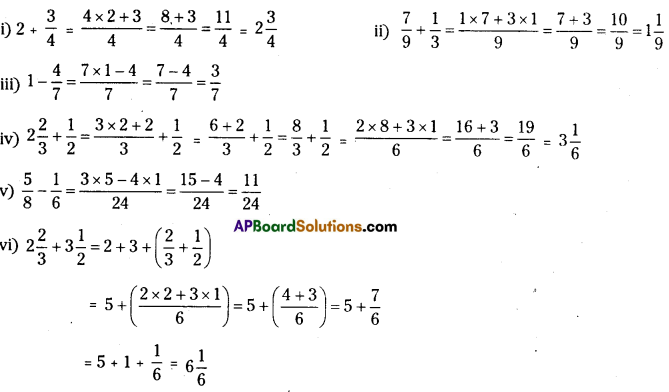Question 2.
Arrange the following in ascending order.
(i) $$\frac{5}{8}, \frac{5}{6}, \frac{1}{2}$$
(ii) $$\frac{2}{5}, \frac{1}{3}, \frac{3}{10}$$
Solution: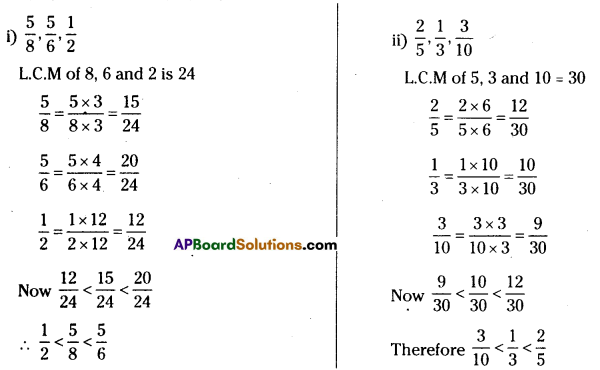Question 3.
Check whether in this square the sum of the numbers in each row and in each column and along the diagonals is the same.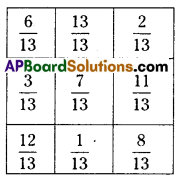Solution: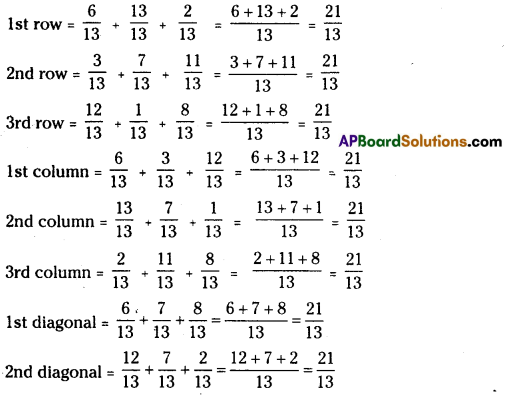Question 4.
A rectangular sheet of paper is 5$$\frac{2}{3}$$ cm long and 3$$\frac{1}{5}$$ cm wide. Find its perimeter.
Solution:
Length of the rectangular sheet = 5$$\frac{2}{3}$$ cm
Breadth/width of the rectangular sheet = 5$$\frac{2}{3}$$ cm
Perimeter = 2 x (length + breadth)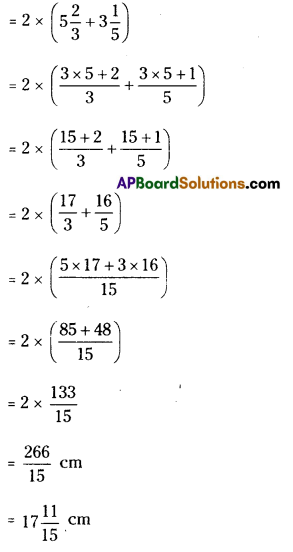Question 5.
The recipe requires 3$$\frac{1}{4}$$ cups of flour. Radha has 1$$\frac{3}{8}$$ cups of flour. How many more cups of flour does she need?
Solution:
Flour required for the recipe = 3$$\frac{1}{4}$$ cups
Flour with Radha = 1$$\frac{3}{8}$$ cups
More cups of flour required = $$3 \frac{1}{4}-1 \frac{3}{8}$$
= $$\frac{3 \times 4+1}{4}-\frac{1 \times 8+3}{8}$$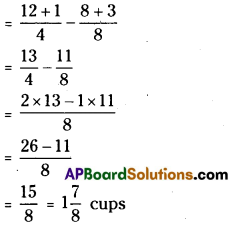Question 6.
Abdul is preparing for his final exam. He has completed $$\frac{5}{12}$$ part of his course content. Find out how much course content is left?
Solution:
Take content as 1 (i.e., full) Course completed = $$\frac{5}{12}$$
Course yet to be completed = 1 – $$\frac{5}{12}$$
= $$\frac{12 \times 1-5}{12}$$
$$\frac{12-5}{12}=\frac{7}{12}$$

Question 7.
Find the perimeters of(i) ΔABE (ii) the rectangle BCDE in this figure. Which figure has greater perimetre and by how much?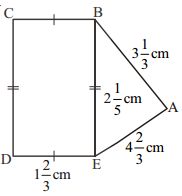Solution:
i) Perimeter of ΔABE = AB + BE + AE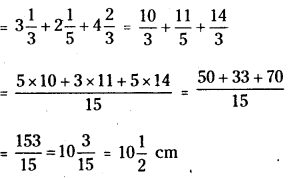ii) Perimeter of BCDE = 2(BE + BC)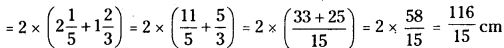As $$\frac{116}{15}<\frac{153}{15}$$, we conclude that the perimetre of ΔABE > Perimeter of BCDE